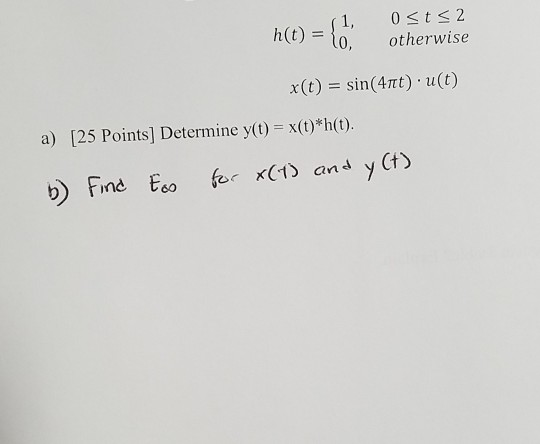Home / Answered Questions / Other / osts2-h-t-0-h-t-1-otherwise-x-t-sin-4ttt-u-t-a-25-points-determine-y-t-x-t-h-t-b-find-eco-for-x-1-an-aw408

# (Solved): Osts2 H(t) = {0, H(t) = {1, Otherwise X(t) = Sin(4ttt)u(t) A) [25 Points] Determine Y(t) = X(t)*h(t)...Osts2 h(t) = {0, h(t) = {1, otherwise x(t) = sin(4ttt)u(t) a) [25 Points] Determine y(t) = x(t)*h(t). b) Find Eco for X(1) and y Ct)

We have an Answer from Expert